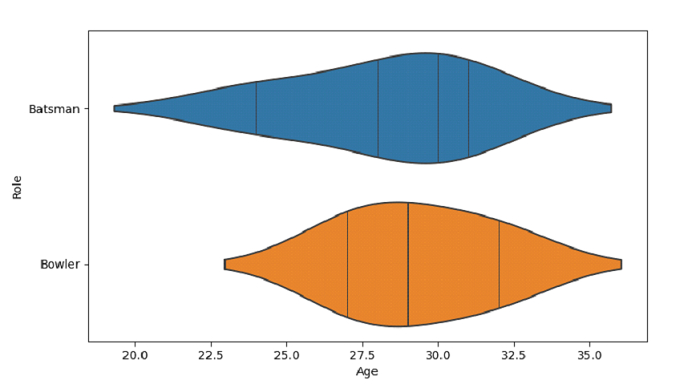# Python Pandas - Plot horizontal violins and order an explicit order with observations in Seaborn

Violin Plot in Seaborn is used to draw a combination of boxplot and kernel density estimate. The seaborn.violinplot() is used for this. Order with order parameter and set observations using inner parameter.

Let’s say the following is our dataset in the form of a CSV file − Cricketers.csv

At first, import the required libraries −

import seaborn as sb
import pandas as pd
import matplotlib.pyplot as plt

Load data from a CSV file into a Pandas DataFrame −

dataFrame = pd.read_csv("C:\Users\amit_\Desktop\Cricketers.csv")


Plot horizontal violin plot with Role and Age columns. Order with order parameter and set observations using inner parameter −

sb.violinplot(x = 'Age', y = "Role", order=["Batsman", "Bowler"],
data = dataFrame, inner="stick")

## Example

Following is the code −

import seaborn as sb
import pandas as pd
import matplotlib.pyplot as plt

# Load data from a CSV file into a Pandas DataFrame:

# plotting violin plot with Role and Age
# order with order parameter and set observations using inner parameter
sb.violinplot(x = 'Age', y = "Role", order=["Batsman", "Bowler"],data = dataFrame, inner="stick")

# display
plt.show()

## Output

This will produce the following output −